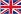English
How to Calculate The Unfolded Length of Groove Bending?
You are here: » » » How to Calculate The Unfolded Length of Groove Bending?

# How to Calculate The Unfolded Length of Groove Bending?

Views: 94     Author: Site Editor     Publish Time: 2023-10-08      Origin: Site

As shown in the figure, planing is now used to bend a 20×20 square tube with a plate thickness of 1mm.The remaining material thickness a of the planing groove is related to the size of the right-angle arc after bending by the customer, and also to the size of the material thickness t. Generally speaking, when the material thickness is less than or equal to 1mm, a=0.4mm, and when the material thickness is greater than 1mm, a= t/2.

L=2×(L1+L2)=2x((side length-1xa-0.2)+(side length-2xa)) of the illustrated example

=2x((20-1×0.4-0.2)+(20-2×0.4))=77.2mm

Note: One end of L1 is planed off, no matter how thick the material is, 0.2mm is deducted

Let's compare the difference between the calculation of bending and unfolding through slotted bending and non-slotted bending:For slotted bends, the method for calculating the total length is:

L=20-0.4+20-2X0.4+20-0.4=58.4

For non-slotted bends, the method for calculating the total length is:

●L=(20-1xt+k)+(20-2xt+2xk)+(20-1xt+1xk)=(20-1+0.2)+(20-2+2×0.2)+(20-1+ 0.2)=56.8mm

●t is the material thickness, k is the coefficient, the size of k is related to the material thickness, generally between 0.2-0.25, the thicker the material, the greater the K value. In this example, k is selected as 0.2.

The following is a combination of a Z-shaped slot and no slot to calculate the unfolded length of the bend:For the 2mm board, the remaining material thickness a of the planing groove is taken as t/2=1mm, (if the customer requires the direct arc to be smaller, the a value should be selected less than 1mm) So the expansion length:

L=15-1+20-2x1+15-1=46mm

For a 2mm thick board, without grooving and bending, the coefficient k is selected as 0.25, so the unfolded length:

L=(15-2+0.25)+(20-2×2+2×0.25)+(15-2+0.25)=43mm

The calculation method is described below with an example of grooving and non-grooving of a multi-bend workpiece:For the 3mm thick plate, it is assumed that the customer requires the right-angle arc to be smaller, then the remaining material thickness is 0.5mm, and the expanded length L=(40-0.5)+(30-2×0.5)+(30-2×0.5) +(10-0.5)=107mm;

For a 3mm thick plate, the coefficient k is selected as 0.25, so the unfolded length:

L=(40-3+0.25)+(30-6+2×0.25)+(30-6+2×0.25)+(10-3+0.25)=93.5mm

The following is an example of reverse bending after grooving to introduce the method of calculating expansion:For back bends after gouging as shown

Calculate the length of the expansion as

L=(20-2+0.2)+(30-2×2+2×0.2)+(20-2+0.2)=62.8mm